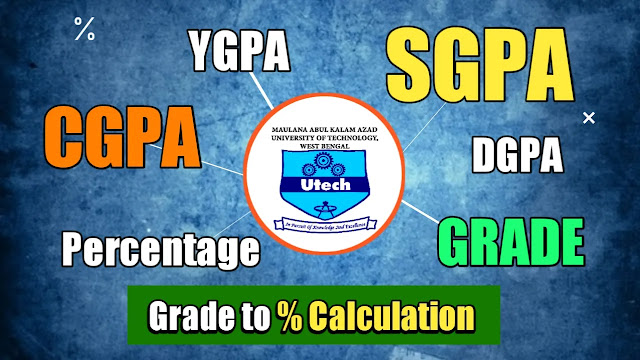MAKAUT CA3 2023 Mandatory (New Rules) View

# How to calculate CGPA in MAKAUT: SGPA,CGPA, DGPA | SGPA to Percent Calculate System

Table of Content

how to calculate cgpa in makaut, makaut dgpa to percentage conversion, how to calculate dgpa in makaut, makaut grading system, how to calculate percentage from dgpa in makaut, dgpa to cgpa  converter, how to calculate total marks in wbut, makaut cgpa to percentage calculatorImage: SGPA,CGPA, DGPA in MAKAUT

Introduction

As a college Student you have always a doubt about Marks Grade System that What is SGPA, CGPA, PGPA, DGPA and many things related this terms.
In today's article I will try to clear your doubt related All this terms and Discuss how to convert Grade to Percent.
Now all College or University (i.e. MAKAUT) publish their result in Grade format.

But in Scholarship, interviews or Competitive Examination is always need a Percent Cut-Off as Result Eligibility. So, Students always have doubt how to Calculate Grade to percentage.

Here is Simple tool for College students Under Makaut for Convert their DGPA /CGPA  grade to Percentage.

DGPA to Percent Covert Tool

MAKAUT SGPA/YGPA to Percent Converter

Enter you SGPA/YGPA/CGPA of a Semester to Calculate your Percentage (%). Also, Put Particular Percentge to Know Exact Grade.

• UCanTool App
• This Tool is for those, who want to calculate their all marks Manually. You can also check any Percentage Cut-off by Converting in Grade Points. It is a Mlti tool, that converts, both Grade ⇉ Percentage(%), as well as Percentage(%) ⇉ Grade Points.SVMCM Marks Calculator Tool for Renewal

SHARE this "SGPA DGPA Marks Percentage Calculator" tool with your Friends and Help them to Calculate their Marks in such simple way. Have a Great Day!!
Overview
• Semester Grade Point Average Rules and Calculation [SGPA]
• Yearly Grade Point Average Rules and Calculation [YGPA]
• Degree Grade Point Average Calculation [DGPA]
• DGPA for 4 year Course
• DGPA for Lateral Entry Course
• DGPA for 3 year Graduation CourseGrade to Percent % Calculation

G* Classification Score on 100 Percentage Points Points
O Outstanding 100 to 90 10
E Excellent

89 to 80

9
A Very Good 79 to 70 8
B Good 69 to 60 7
C Fair 59 to 50 6
D Below Average

49 to 40

5
F Failed Below 40 2
I Incomplete --- 2

Letter Grades and their corresponding classification and percentage points
Article Topic Covered:
• how to calculate cgpa in makaut
• makaut dgpa to percentage conversion
• how to calculate dgpa in makaut
• how to calculate percentage from dgpa in makaut
• dgpa to cgpa converter
• how to calculate total marks in wbut
• makaut cgpa to percentage calculator